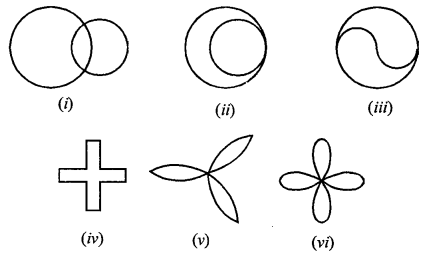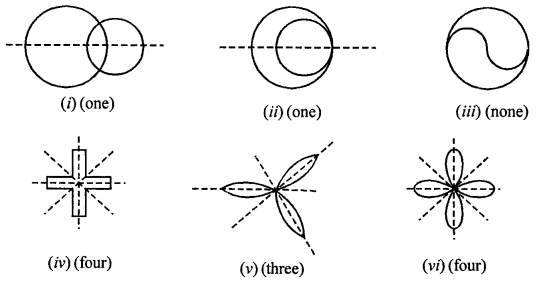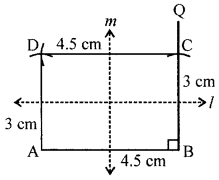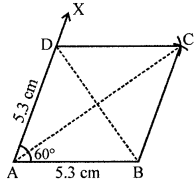## ML Aggarwal Class 8 Solutions for ICSE Maths Chapter 16 Symmetry Reflection and Rotation

Question 1.
Draw the line or lines of symmetry, if any, of the following shapes and count their number :Solution:Question 2.
For each of the given shape in question 1, find the order of the rotational symmetry (if any).
Solution:
(i) None
(ii) None
(iii) Two
(iv) Four
(v) Three
(vi) Four

Question 3.
Construct a rectangle ABCD such that AB = 4.5 cm and BC = 3 cm. Draw its line (or lines) of symmetry.
(i) Draw a line AB = 4.5 cm.
(ii) Draw a line BQ from point B making an angle of 90°.
(iii) Taking B as centre and radius = 3 cm,
mark an arc on BQ, name it as C.
(iv) As we know, opposite sides of rectangle are equal.
Therefore from point C. As a centre and radius = 4.5 cm,
draw an arc and with A as centre and 3 cm as radius,
make another as C, cutting the previous arc at point D.
(v) Join DC and DA.
(vi) ABCD is a required rectangle.
Lines of symmetry:It has 2 lines of symmetry,
the lines joining the midpoints of opposite sides.

Question 4.
Construct a rhombus ABCD with AB = 5.3 cm and ∠A = 60°. Draw its line (or lines) of symmetry.
Solution:
(i) Draw line segment AB = 5.3 cm.
(ii) Construct ∠BAX = 60°.
(iii) With A as centre, radius = 5.3 cm draw an arc on AX.
(iv) Mark the point of intersection = D.
(v) With D as the centre and radius = 5.3 cm, draw an arc.
(vi) With B as centre and same radius,
draw another arc, such that it intersects the previous at C.
(vii) Join CD and CB.
ABCD is a required rhombus.Lines of symmetry in rhombus = 2,
the diagonals of the rhombus.